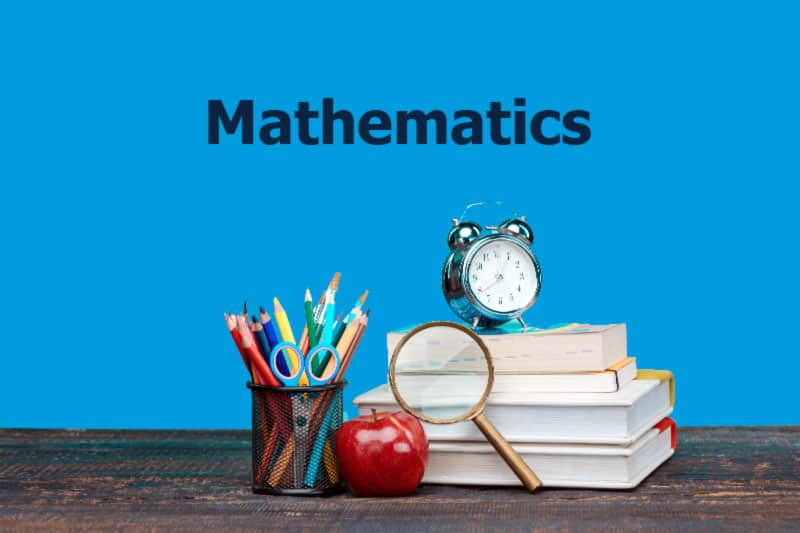# Mathematics Curriculum Guide

The study of mathematics is a way to investigate and appreciate order in God’s creation. Using a wide variety of tools, teachers can guide students in developing a deep conceptual understanding in order to make sense of mathematics. Due to the ever-increasing scientific and technological developments of our age, students will master the specific knowledge necessary for its application to real problems, for the study of related subject matter, and for continued study in mathematics. The mathematics program will provide our students with the learning opportunities to develop the skills, positive attitudes, and abilities needed to function well in daily Christian living.

### Objectives

Preschool – initial recognition of numbers and quantity

Kindergarten – representing and comparing whole numbers / describing shapes and space

Grade 1 – developing understanding in addition and subtraction/ whole number relationships and place value / developing understanding of linear measurement/ reasoning about geometric shapes

Grade 2 – base-ten notation / fluency with addition and subtraction / units of measure / describing and analyzing shapes

Grade 3 – developing understanding of multiplication and division strategies / developing understanding of unit fractions / developing understanding of rectangular arrays and area / describing and analyzing two-dimensional shapes

Grade 4 – developing understanding of multi-digit multiplication and division / developing understanding of fractional equivalence / and geometric shape analyzation

Grade 5 – developing fluency of all 4 operations with fractions / understanding of place values and operations with decimals / developing understanding of volume

Grade 6 – developing understanding of ratio and rate / completing understanding of division with fractions / introduction of negative numbers / use of expressions and equations / developing understanding of statistical thinking

Grade 7 – developing understanding of proportional relationships / expressions and linear equations / problem solving with scale drawings, constructions, multidimensional shapes, area, surface area, and volume / and making inferences based on data and the use of samples

Grade 8 – formulating and reasoning about expressions and equations by using linear equations and systems of linear equations / using functions to describe a quantitative relationship / analyzing 2- and 3-dimensional space / apply the Pythagorean Theorem 8 – Algebra seeing structure in expressions and equations / arithmetic with polynomials and rational functions / create and write equations / develop reasoning with equations and inequalities